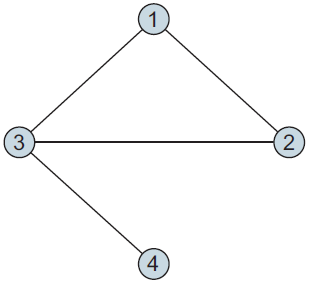## Spark GraphX之全局聚类系数、局部聚类系数、网络平均聚类系数 原

### Global clustering coefficient（全局聚类系数）

Another way to measure connectedness, the global clustering coefficient, is better in that it always returns a number between 0 and 1, making it possible to compare the connectedness of different sized graphs.

A triplet in this case is a set of three vertices that have two or three edges among them. If there are three edges, then it’s a triangle, and this is called a closed triplet. If there are only two edges, then it’s called an open triplet. Triplets are counted for each vertex and then added all together; this means that a triangle will count as three closed triplets, because each of the three vertices will have one closed triplet associated with it.``````  def globalClusteringCoefficient[VD: ClassTag, ED: ClassTag](g:Graph[VD, ED]) = {
val numerator  = g.triangleCount().vertices.map(_._2).reduce(_ + _)
val denominator = g.inDegrees.map{ case (_, d) => d*(d-1) / 2.0 }.reduce(_ + _)
if(denominator == 0) 0.0 else numerator / denominator
}
``````

### local clustering coefficient（局部聚类系数）

that is the ratio of the actual triangle count at a vertex to the number of possible triangles at that vertex based on how many neighbors it has. For an undirected graph, the local clustering coefficient C for a vertex that has k neighbors and t triangles is: C = 2t / [ k * (k - 1) ]

``````  def localClusteringCoefficient[VD: ClassTag, ED: ClassTag](g: Graph[VD, ED]) = {
val triCountGraph = g.triangleCount()
val maxTrisGraph = g.inDegrees.mapValues(srcAttr => srcAttr*(srcAttr-1) / 2.0 )
triCountGraph.vertices.innerJoin(maxTrisGraph){ (vid, a, b) => if(b == 0) 0 else a / b }
}
``````

### network average clustering coefficient（网络平均聚类系数）

Computing the average value of the local clustering coefficient for all of the vertices in the graph gives us the network average clustering coefficient

``````    val vertices = sc.makeRDD(Seq((1L, ""), (1L, ""), (2L, ""), (3L, ""), (4L, ""), (4L, "")))
val edges = sc.makeRDD(Seq(Edge(1L, 1L, ""), Edge(1L, 2L, ""), Edge(2L, 3L, ""), Edge(1L, 3L, ""), Edge(3L, 4L, ""))).filter(e => e.srcId != e.dstId).flatMap(e => Array(e, Edge(e.dstId, e.srcId, e.attr))).distinct()  //去掉自循环边，有向图变为无向图，去除重复边
val testGraph =  Graph(vertices, edges).cache()
``````

``````    testGraph.vertices.foreach(println)
``````

``````(3,)
(1,)
(2,)
(4,)
``````

The vertices and edges arguments that we used to construct the Graph instance were regular RDDs—we didn’t even deduplicate the entries in the vertices so that there was only a single instance of each topic. Fortunately, the Graph API does this for us, converting the RDDs we passed in to a VertexRDD and an EdgeRDD, so that the vertex counts are now unique. Note that if there are duplicate entries in the EdgeRDD for a given pair of vertices, the Graph API will not deduplicate them: GraphX allows us to create multigraphs, which can have multiple edges with different values between the same pair of vertices. This can be useful in applications where the vertices in the graph represent rich objects, like people or businesses, that may have many different kinds of relationships between them (e.g., friends, family members, customers, partners, etc.). It also allows us to treat the edges as either directed or undirected, depending on the context.

``````    println(globalClusteringCoefficient2(testGraph))
``````

``````0.6
``````

``````    localClusteringCoefficient(testGraph).foreach(println)
``````

``````(3,0.3333333333333333)
(1,1.0)
(2,1.0)
(4,0.0)
``````

``````    println(localClusteringCoefficient(testGraph).map(_._2).sum() / testGraph.vertices.count())
``````

``````0.5833333333333333
``````

© 著作权归作者所有### 绝世武神

Spark 数据分析导论-笔记

Spark Core Spark Core 实现了Spark 的基本功能，包含任务调度、内存管理、错误恢复、与存储系统交互等模块。 Spark Core 中还包含了 对弹性分布式数据集（resilient distributed dataset，简...

Java搬砖工程师
2018/12/26
28
0
Spark GraphX宝刀出鞘，图文并茂研习图计算秘笈与熟练的掌握Scala语言【大数据Spark

Spark GraphX宝刀出鞘，图文并茂研习图计算秘笈 大数据的概念与应用，正随着智能手机、平板电脑的快速流行而日渐普及，大数据中图的并行化处理一直是一个非常热门的话题。图计算正在被广泛地...

Spark亚太研究院
2014/08/29
1K
0
Spark2.1.0之模块设计

2018/06/05
0
0
[Kafka与Spark集成系列一] Spark入门

2018/08/26
0
0
Spark之GraphX的特点

1.基于内存实现了数据的复用与快速读取 具有较多迭代次数是图计算算法的一个重要特点。在海量数据背景下，如何保证图计算算法的执行效率是所有图计算模型面对的一个难题。基于MapReduce的图计...

mmake1994
2018/04/16
0
0

OSChina 周六乱弹 —— 早上儿子问我他是怎么来的

Osc乱弹歌单（2019）请戳（这里） 【今日歌曲】 @凉小生 ：#今日歌曲推荐# 少点戾气，愿你和这个世界温柔以待。中岛美嘉的单曲《僕が死のうと思ったのは (曾经我也想过一了百了)》 《僕が死の...

1K
12
Excption与Error包结构，OOM 你遇到过哪些情况，SOF 你遇到过哪些情况

Throwable 是 Java 中所有错误与异常的超类，Throwable 包含两个子类，Error 与 Exception 。用于指示发生了异常情况。 Java 抛出的 Throwable 可以分成三种类型。 被检查异常（checked Exc...

Garphy

38
0

FAT_mt

40
0

61
0
tabel 中含有复选框的列 数据理解

1、el-ui中实现某一列为复选框 实现多选非常简单: 手动添加一个el-table-column，设type属性为selction即可； 2、@selection-change事件：选项发生勾选状态变化时触发该事件 <el-table @sel...

everthing

20
0